Courses

# NTSE Level Test: Periodic Classification Of Elements

## 20 Questions MCQ Test Science Class 10 | NTSE Level Test: Periodic Classification Of Elements

Description
This mock test of NTSE Level Test: Periodic Classification Of Elements for Class 10 helps you for every Class 10 entrance exam. This contains 20 Multiple Choice Questions for Class 10 NTSE Level Test: Periodic Classification Of Elements (mcq) to study with solutions a complete question bank. The solved questions answers in this NTSE Level Test: Periodic Classification Of Elements quiz give you a good mix of easy questions and tough questions. Class 10 students definitely take this NTSE Level Test: Periodic Classification Of Elements exercise for a better result in the exam. You can find other NTSE Level Test: Periodic Classification Of Elements extra questions, long questions & short questions for Class 10 on EduRev as well by searching above.
QUESTION: 1

### Which of the following elements would lose an electron easily ?

Solution:

As we move down a group in the periodic table, the tendency to lose electron increases.
Also when we move from left to right in a period, the tendency to lose electrons decreases. Combining these two results, K will lose the electron most easily.

QUESTION: 2

### Which of the following is non-metal and to which group does it belong?

Solution:

Bromine is a non-metal, belongs to group 17.

QUESTION: 3

### Which one of the following depict the correct representation of atomic radius (r) of an atom ?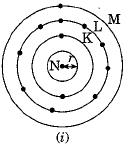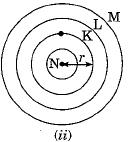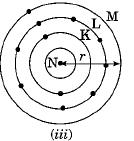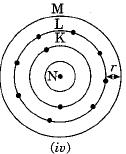Solution:

Atomic radius is the distance between the centre of the nucleus and the outermost shell which contains electrons in an isolated atom. Figure (ii) and (iii) are the correct representation of atomic radius from nucleus to the outermost shell.

QUESTION: 4

Silicon (14) belongs to class of

Solution:

It is metalloid.

QUESTION: 5

According to Mendeleev’s Periodic Law, the elements were arranged in the periodic table in the order of

Solution:

According to Mendeleev's periodic law , the properties of an elements are the function of their atomic masses...

This means that when elements are arranged in the order of increasing number of atomic masses , the properties of elements repeats after a period or intervals...

QUESTION: 6

Chlorine (17) belongs to which group and period

Solution:

Cl (17) 2,8,7 belongs to group 17 and 3rd period.

QUESTION: 7

Which of the following statement(s) about Modern Periodic Table are incorrect ?
(i) The elements in the Modem Periodic Table are arranged on the basis of their decreasing atomic number.
(ii) The elements in the Modem Periodic Table are arranged on the basis of their increasing atomic masses.
(iii) Isotopes are placed in adjoining group(s) in the Periodic Table.
(iv) The elements in the Modern Periodic Table are arranged on the basis of their increasing atomic number.

Solution:

Only the statement (iv) that in the Modern Periodic Table, the elements are arranged on the basis of increasing atomic number.

QUESTION: 8

Which of the following is most reactive

Solution:

F2 is most reactive because it is smaller in size and can gain electron easily.

QUESTION: 9

The concept of triads in the elements was given by

Solution:

Dobereiner gave the concept of triads.

QUESTION: 10

Which of the following is most reactive

Solution:

K is most reactive due to larger size, can lose electron easily

QUESTION: 11

Upto which element, the Law of Octaves was found to be applicable ?

Solution:

The Law of Octave was found to be applicable upto calcium as after that every eighth element did not possess properties of the first element.

QUESTION: 12

Which of the following set of elements is written in order of their decreasing metallic character ?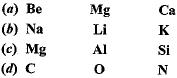Solution:
QUESTION: 13

The elements A, B, C, D and E have atomic number 9, 11, 17, 12 and 13 respectively. Which pair of elements belong to the same group ?

Solution:

Elements A and C belong to the same group because there is a difference o f 8 in the atomic number (F and Cl).

QUESTION: 14

Which one of the following does not increase while moving down the group of the periodic table ?

Solution:
QUESTION: 15

Which of the following statements is not a correct statement about the trends when going from left to right across the periods of periodic table ?

Solution:

The atoms lose the electrons less easily as we move from left to right in the periodic table because metallic character decreases.

QUESTION: 16

The element with atomic number 14 is hard and forms acidic oxide and a covalent halide. To which of the following categories does the element belong?

Solution:
QUESTION: 17

Element X forms a chloride with formula XCl2 which is a solid with a high melting point. X would most likely be in the same group of the Periodic Table as ​

Solution:

XCl2 indicates that it has a valency equal to 2. Magnesium and other elements in this group have the valency equal to 2.

QUESTION: 18

Which of the following elements will form an acidic oxide ?

Solution:
QUESTION: 19

In Mendeleev’s Periodic Table, gaps were left for the elements to be discovered later. Which of the following elements found a place in the periodic table later ?

Solution:

Germanium was discovered later.

QUESTION: 20

Which of the following elements does not lose an electron easily ?

Solution: